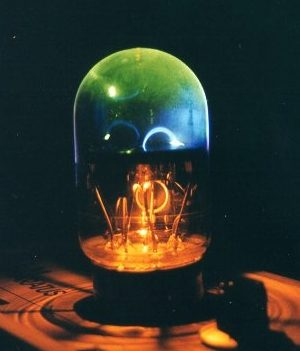### You can bend a beam of electrons with an applied magnetic field to make a great demonstration of the Lorentz Force.

WATCH VIDEO

Teachable Topics:

• Magnetic fields
• Centripetal force
• Kinetic energy

Theory:

Recall from your study of introductory mechanics that when a particle moves along a circular path it is constantly accelerating, and there must be a centripetal force producing this acceleration. This force is given by the following equation:

F= mv2/r

Where m is the particle's mass, v is its speed, and r is the radius of its circular path. By applying a magnetic field of strength B perpendicular to the velocity of the moving electrons, we will produce a centripetal force of strength

F = evB

Where e is the charge of a single electron. By combining the two equations above, we get the following expression for the charge over mass ratio of the electron.

e/m = v/Br

In this experiment, the electrons will be injected into the magnetic field by first accelerating them through a potential difference V. Assuming the electron is at rest when it enters the accelerating region, the electric potential energy when the electron enters the region must equal the kinetic energy when the electron leaves the region. In other words,

eV = 1/2 mv2

Putting this equation into the one previous to it yields the following expression which gives the e/m ratio in terms of the accelerating voltage and the applied magnetic field and the radius of circulating electron's path:

e/m = 2V/B2r2

The magnetic field and the accelerating potential can be manipulated independently, while the radius of the beam's path depends on both V and B. By thoughful experimentation, you can determine the e/m ratio.

Apparatus:

• XH R 60-10 DC power supply
• 620 9 B DC power supply
• E/M apparatus

Procedure:

• Connect and plug in the apparatus. Make sure that the outputs of the power supplies are set to zero before turning them on.
• With no current going through the magnet coils (i.e., Helmholtz coils), apply the acelerating potential so that the electron beam moves up in a vertical direction. Adjust the grid voltage to focus the beam.
• Applying the magnetic field will bend the electron beam.
• The more current that goes through the magnet coils, the more tightly the beam will bend

You can use the magnetic field to bend the beam to a specific radius (one of the concentric circles in the vacuum tube). You'll know the beam is hitting one of the radius' because you'll see a green glow from the phosphorescent coating on the lines• Reverse the current through the magnetic field to "flip" the electron beam over. Doing a measurement at a particular radius, then "flipping" the beam to hit the same radius on the other side is a way to get more accurate data. We recommend using the average of the magnetic field measurments required to hit the same circle as a best-measure of the field required for that radius.
• Be sure to note uncertainties in potentials and currents, and to deal with the uncertainty in beam position

SAFETY WARNINGS!

• Please be sure to note any warnings of maximum current/voltage load on the main apparatus. It is possible to burn out the coils and damage the apparatus